## Monday, August 31, 2009

### 974

974 = 2 x 487.

974! – 1 is a factorial minus one prime.

974 is the number of multigraphs with 5 vertices and 10 edges.

974 is the sum of six positive fifth powers: 974 = 35 + 35 + 35 + 35 + 15 + 15.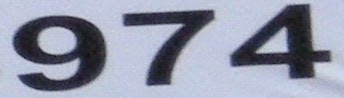974 was the year of a great earthquake in England.

## Friday, August 28, 2009

### 867

867 = 3 x 172

867 is the number of graphs with 8 vertices that have chromatic number 5.

867 is a number n such that n times n + 7 gives the concatenation of two numbers m and m + 1: 867 x 874 = 757758.

867 is a number such that the sum of its digits is seven times the number of digits: 8 + 6 + 7 = 21 = 3 x 7."867-5309/Jenny" is a song written by Alex Call and Jim Keller and performed by Tommy Tutone. It peaked at number four on the Billboard Hot 100 chart and number one on the Billboard Top Tracks chart in 1982.

## Thursday, August 27, 2009

### 751

751 is a prime number.

751 is the largest prime that cannot be expressed as the sum of five or fewer squared composite numbers.

751 is the smallest integer with the following property: the sum of digits of 751 = the sum of digits of the 751st prime = the sum of digits of the 751st semiprime (product of two prime numbers).

The odd powers of 751 end with 751; and its even powers end with 001.751 Magazine is an online magazine for poetry and short fiction.

Source: Prime Curios!

## Wednesday, August 26, 2009

### 630

630 = 2 x 32 x 5 x 7.

630 is a triangular number. It is the sum of the first 35 integers: 1 + 2 + 3 + . . . + 34 + 35 = 630. 630 is also three times a triangular number (210) and six times a triangular number (105).

630 is 21312 in base 4.

630 is the sum of six consecutive primes: 97 + 101 + 103 + 107 + 109 + 113 = 630.630 is the height and the span (in feet) of the stainless steel arch “Gateway to the West” in St. Louis, Missouri.

## Tuesday, August 25, 2009

### 514

514 = 2 x 257.

514 is a centered triangular number.

514!/415! + 1 is a prime number.

514 is 20002 in base 4, 4024 in base 5, 1333 in base 7, and 1002 in base 8.514 is a telephone area code that covers all of the island of Montreal, Quebec.

Source: Prime Curios!

## Monday, August 24, 2009

### 411

411 = 3 x 137.

The polynomial 30x - 13 produces 411 primes for the first 1000 values of x.

411 is 12123 in base 4.

411 is a member of the Fibonacci-type sequence starting with 1 and 4.411 is the telephone number within the U.S. for information or directory services.

Source: Prime Curios!

## Friday, August 21, 2009

### 301

301 = 7 x 43.

301 is the product of the only two primes (7 and 43) that can be the last two digits of a fifth power.

301 is the maximum number of regions into which 24 lines divide a plane. It is a lazy caterer’s number. The lazy caterer's sequence describes the maximum number of pieces of a circle (pizza) that can be made with a given number of straight cuts.

3012 = 90601 and 1032 = 10609.

301 is the sum of three consecutive primes: 97 + 101 + 103 = 301.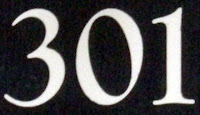301 is the telephone area code for parts of Maryland.

Source:
Wikipedia

## Thursday, August 20, 2009

### 299

299 = 13 x 23.

299 is the smallest number whose sum of digits is 20.

299 is a cake number. A cake number is the maximum number of pieces into which a 3-dimensional space can be cut with a given number of plane cuts: 12 cuts produce 299 pieces.

There are
299 composite numbers less than 1000 that are products of two primes.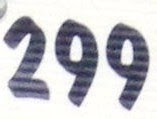299 Thora is a main belt asteroid discovered in 1890 by Johann Palisa in Vienna.

Source:
Wikipedia

## Wednesday, August 19, 2009

### 190

190 = 2 x 5 x 19.

190 has a unique representation as a sum of three squares: 190 = 32 + 92 + 102.

190 is the 19th triangular number; it is the sum of the first nineteen integers: 1 + 2 + 3 + . . . + 18 + 19 = 190.

190 is the largest number with only distinct prime Roman numeral palindrome factors that is itself a Roman numeral palindrome: 190 = CXC = II * V * XIX.

190 is 2332 in base 4 and 1230 in base 5.190 is the sum of all the vertices, edges, and faces of the five Platonic solids.

Source:
Prime Curios!

## Tuesday, August 18, 2009

### 45

45 = 32 x 5.

45 is the ninth triangular number; it is the sum the first nine integers: 1 + 2 + 3 + 4 + 5 + 6 + 7 + 8 + 9 = 45.

45 is the only number that is the sum of its digits multiplied by 5.

If the first five powers of two are each subtracted from 45, all the results are prime: 45 – 2 = 43; 45 – 4 = 41; 45 – 8 = 37; 45 – 16 = 29; 45 – 32 = 11.The chemical element rhodium has atomic number 45.

Source: Number Gossip

## Monday, August 17, 2009

### 493

493 = 17 x 29.

The first few numbers not known to produce palindromes when applying the 196-algorithm (that is, a reverse-then-add sequence) are sometimes known as Lychrel numbers. The first few Lychrel numbers are 196, 295, 394, 493, 592, 689, 691, 788, 790, 879, 887, . . . : 887 = 196 + 691 = 295 + 592 = 394 + 493 = 790 + 097.

493 is 13231 in base 4.

493 is the sum of seven consecutive primes: 59 + 61 + 67 + 71 + 73 + 79 + 83 = 493.493 is the number of Pokémon species, from the first set through the fourth generation.

Source: Wolfram MathWorld

## Friday, August 14, 2009

### 1517

1517 = 37 x 41 (the product of two consecutive prime numbers).

1517 has two representations as a sum of two squares: 1517 = 192 + 342 = 262 + 292.

1517 is the hypotenuse of two primitive Pythagorean triples: 15172 = 1652 + 15082 = 7952 + 12922.

The sum of the decimal digits of 21517 is also a power of 2.

1517 divides the sum of the first 1517 Fibonacci numbers.

1517 was the record-breaking attendance at MAA’s MathFest 2009 in Portland, Ore.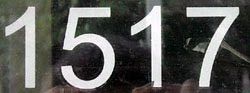Italian mathematician and Franciscan friar Luca Pacioli died in the year
1517.

Source:
Wikipedia

## Thursday, August 13, 2009

### 290

290 = 2 x 5 x 29.

290 equals the tenth prime times ten: 29 x 10 = 290.

290 is the sum of four consecutive primes: 67 + 71 + 73 + 79 = 290.

290 has a base 3 representation (101202) that ends with its base 6 representation (1202).

290 has two representations as a sum of two squares: 290 = 12 + 172 = 112 + 132.NGC
290 is an open cluster of stars in the Small Magellanic Cloud, which is located in the constellation Tucana.

Source:
Wikipedia

## Wednesday, August 12, 2009

### 172

172 = 22 x 43.

172 has a unique representation as a sum of three squares: 172 = 62 + 62 + 102.

172 is 444 in base 6.

Each internal angle of a regular 45-sided polygon is
172o.

172 is the maximum number of regions into which 18 lines divide the plane. Hence, 172 is a member of the lazy caterer's sequence, which describes the maximum number of pieces of a circle (a pancake or pizza) that can be made with a given number of straight cuts. For example, three cuts across a pancake will produce six pieces if the cuts all meet at a common point, but up to seven if they do not.Ethanol (ethyl alcohol) boils at
172 oF under standard conditions.

Source:
Wikipedia

## Tuesday, August 11, 2009

### 44

44 = 22 x 11.

44 is the smallest number that is the sum of a reversible pair of non-palindromic primes: 13 + 31 = 44.

44 is the first number such that it and the next number are the product of a prime and another distinct prime squared: 44 = 22 x 11 and 45 = 32 x 5.

44 is the maximum number of regions into which the plane can be divided by seven circles.44 is the atomic number of the chemical element ruthenium.

Source: Number Gossip

## Monday, August 10, 2009

### 1101

1101 = 3 x 367.

1101 has a base 2 representation (10001001101) that ends in 1101.

1101 is the sum of three distinct powers of 10: 1101 = 103 + 102 + 100.

1101 is a number divisible by the sum of the squares of its digits.1101 Clematis is an asteroid discovered in 1928 and named for a flower.

## Friday, August 7, 2009

### 900

900 = 22 x 32 x 52.

900 is a square number: 30 x 30 = 900.

900 is the smallest square that is the sum of different primes by using all ten digits: 900 = 503 + 241 + 89 + 67 = 509 + 283 + 61 + 47.

900 has a base 5 representation (12100) that begins with its base 9 representation (1210). Its base 7 representation is 2424.

900 has a representation as a sum of two squares: 900 = 182 + 242.900 is the sum (in degrees) of the internal angles of a heptagon.

Source: Prime Curios!

## Thursday, August 6, 2009

### 894

894 = 2 x 3 x 149.

894 has a base 5 representation (12034) that begins with its base 9 representation (1203).

894 is the smallest of three consecutive integers divisible by three consecutive primes respectively: 894 is divisible by 3, 895 is divisible by 5, and 896 is divisible by 7.

894 is the sum 13 positive fifth powers.Interstate 894 is a stretch of highway that serves as a bypass for Milwaukee, Wisconsin.

## Wednesday, August 5, 2009

### 784

784 = 24 x 72.

784 is a square number: 28 x 28 = 784.

784 is the square of the sum of the first seven integers: (1 + 2 + 3 + 4 + 5 + 6 + 7)2 = 784.

784 is the sum of the first seven cubes: 13 + 23 + 33 + 43 + 53 + 63+ 73 = 784.

784 is 1002001 in base 3 and 3344 in base 6.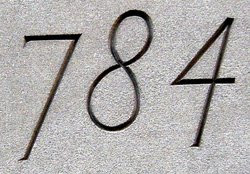Asteroid 784 Pickeringia was discovered in 1914.

Source: Numeropedia

## Tuesday, August 4, 2009

### 638

638 = 2 x 11 x 29.

638 is the sum of four consecutive primes: 151 + 157 + 163 + 167 = 638.

638 is the solution to the postage stamp problem for 7 denominations and 6 stamps.638 is a centered heptagonal number.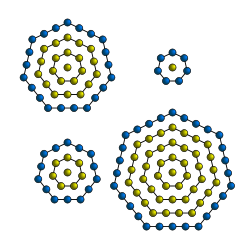The Islamic calendar was introduced in the year 638.

Source: Wikipedia

## Monday, August 3, 2009

### 526

526 = 2 x 263.

526 is the number of ways to cut an 8-by-8 chessboard into two pieces with equal areas using a cut that travels only up and right.

526 is a Smith number. It a composite number such that the sum of its digits is equal to the sum of its prime factors: 5 + 2 + 6 = 2 + 2 + 6 + 3 = 13.

526 and 527 are both the products of two primes: 526 = 2 x 263 and 527 = 17 x 31.526 is a centered pentagonal number.